# Scattering Cross Section

The concept of cross section, as its name suggests, is that of effective area for collision. The cross section of a spherical target is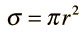Discussion of cross section

In aiming a beam of particles at a target which is much smaller than the beam, as in the Rutherford scattering experiment, the cross section takes on a statistical nature.

Rutherford worked out the scattering cross section for alpha particles of kinetic energy KE scattering off a single nucleus with atomic number Z. The cross section for scattering at a greater angle than some chosen angle is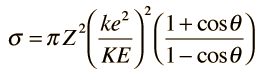Show

More detail is needed for the prediction of the number of scattering events to expect above that angle. When the details about the beam and target are included, the fractional scattering rate is given by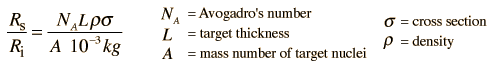The process for dealing with multiple targets is illustrated below.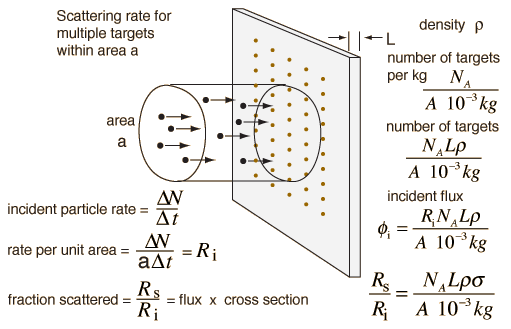For an experiment where projectiles of charge Z = and kinetic energy KE = MeV
are scattered by nuclei of atomic number Z= and mass number A = ,
the scattering cross section is fm2 = barns.

If the scattering target is in the form of a thin foil of thickness L = x 10^m
with density = gm/cm3,
the scattered fraction for angles greater than degrees
is expected to be x10^ .

This calculation is designed for the calculation of cross section and scattered fraction only. Note that the standard assumptions of Rutherford scattering apply, so that the calculation works only for film thicknesses of a few micrometers or less. Default values will be entered for unspecified parameters, but all values except the cross section and scattered fraction can be changed.

 Numerical example: gold foil
Index

Rutherford concepts

 HyperPhysics***** Nuclear R Nave
Go Back

# Scattering Cross Section

The concept of cross section, as its name suggests, is that of effective area for collision. The cross section of a spherical target isThe units of cross section are then area units, but for nuclear scattering the effective area is on the order of the cross sectional area of a nucleus. For a gold nucleus of mass number A=197, the radius determined from the nuclear radius relationship is about 7 fermis.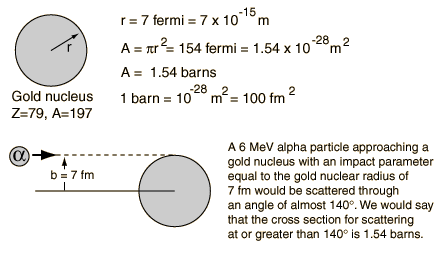The most common unit for cross section for nuclear scattering interactions is the barn. The cross section in barns for alpha scattering above a selected angle is a standard part of the analysis of Rutherford scattering. In the case of 6 MeV alpha particles scattered from a gold foil, for example, you don't know the impact parameter for any given alpha particle, so the calculation of the scattered fraction takes on a statistical character. For alpha particles bombarding a gold foil of thicknes 1 micrometer, less than 1 in 100,000 would meet the conditions for scattering at over 140°. The cross section for scattering at smaller angles will be larger, because the alpha doesn't have to come as close to be scattered through the smaller angle. For example, the cross section for scattering through 90° or above is 11.3 barns.

For neutron scattering, there is no coulomb repulsion, so there is no appreciable scattering unless the impact parameter is less than the effective radius for the strong interaction. Therefore for a given target geometry the cross sections are smaller, typically measured in millibarns.

Since the probability for interaction depends upon the strength and range of the interaction, the cross-sections for neutrino interactions are very small since they interact only by the weak interaction.

 Numerical example: gold foil
 At what thickness do you have to be concerned about multiple scattering?
Index

Rutherford concepts

 HyperPhysics***** Nuclear R Nave
Go Back